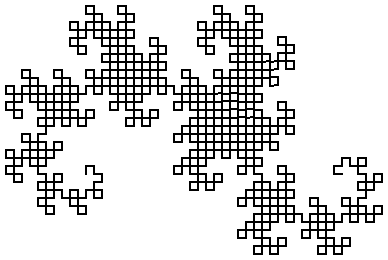7.8

### 7Simplified Lindenmayer System Language

 #lang lindenmayer/simple package: lindenmayer

The lindenmayer/simple language is a trimmed down version of #lang lindenmayer that supports only a single Lindenmayer system and does not support parametric or conditional Lindenmayer systems, nor does it support interoperability with other languages. It is intended to be a digestible example of a language implemented with #lang.

Here is one example use of the language.

 #lang lindenmayer/simple ## axiom ## A ## rules ## A -> AB B -> A ## variables ## n=3

When it is run, it produces the output ABAAB.

There are three main pieces to the implementation of the language: the parser, which translates the notations above into a use of lindenmayer-system, the macros that translate that into a call to the run-lindenmayer function, and then that function itself.

#### 7.1The Parser

 (require lindenmayer/simple/parse) package: lindenmayer

 procedure(parse-module port name) → syntax? port : input-port? name : any/c
Parses a Lindenmayer system program from port, treating name as the the location of the port’s content.

The resulting syntax object is a module form that contains a call to lindenmayer-system. For example, the result of calling parse-module on the example above produces a syntax object whose shape (when stripped of all lexical content and source location information) is this expression:
 '(module name racket/base (require lindenmayer/simple/compile) (define (finish val) (newline)) (define (A value) (display 'A)) (define (B value) (display 'B)) (lindenmayer-system (void) finish 3 (A) (A -> A B) (B -> A)))

#### 7.2The Compiler

 (require lindenmayer/simple/compile) package: lindenmayer

syntax

 (lindenmayer-system start-expr finish-expr iterations-expr axiom rule ...)

 axiom = (id ...) rule = (id -> id ...) | (id → id ...)

 start-expr : any/c
 finish-expr : (-> any/c any/c)
 iterations-expr : natural?
Runs the given Lindenmayer system, where the axiom and rules are sequences of identifiers that are expected to be bound to procedures that accept one argument. Once the Lindenmayer system completes, the procedures are called on the resulting string, in the order of the identifiers in the string, and are used to read a result out of the lindenmayer system.

This form is implemented by compiling into a call to run-lindenmayer.

This example starts from A, iterates the Lindenmayer system 3 times to arrive at the string ABAAB. Then it traverses the string making calls to the procedures A and B in the given order. So, first it calls A with '(), which returns (cons 'A '()), and so forth. Finally, it calls reverse on the elements of the list.
(define (A lst) (cons 'A lst))

(define (B lst) (cons 'B lst))

 > (lindenmayer-system '() reverse 3 (A) (A -> A B) (B -> A))

'(A B A A B)

Instead of just pulling the string back out of the system, we can use turtle graphics to extract a picture:
(define (X t) t)

(define (Y t) t)

(define (F t) (draw 4 t))

(define ( t) (turn -90 t))

(define ( t) (turn 90 t))

 > (lindenmayer-system (turn 90 (turtles 100 100)) turtles-pict 10 (F X) (X -> X ↰ Y F ↰) (Y -> ↱ F X ↱ Y))#### 7.3The Runtime

 (require lindenmayer/simple/run) package: lindenmayer

This module is implemented in Typed Racket, so its inputs are described as types. (Typed Racket can be called from Racket, and vice-versa.)

 type

struct

 (struct cell (item)
#:extra-constructor-name make-cell
#:mutable)
item : (Lindenmayer-Dag α)
The Lindenmayer-Dag and cell types are the runtime representation of the Lindenmayer system as it evolves. Roughly, they are trees with lists of children at each interior node. The leaves are procedures that are used when the final tree has been constructed to extract the value. The trees are actually DAGs and the sharing is used to get better performance while building the final string. Once that final string is constructed, the extraction process traverses the DAG, calling each of the procedures in the leaf nodes.

The (Lindenmayer-Dag α) type is equivalent to
 (U (-> α α) (Listof (cell α)))

procedure

 (run-lindenmayer iterations axiom nts rules init) → α
iterations : Natural
axiom : (cell α)
nts : (Listof (cell α))
rules :
 (Listof (-> (Listof (cell α)) (Listof (cell α))))
init : α
Implements the runtime support for evaluating a Lindenmayer system.

The axiom is expected to be an interior node whose children are all leaves (and thus contain procedures). The nts and the rules arguments are expected to have the same length; each pair of elements (one from each argument) together represent a single rule. When performing a rewriting step, run-lindenmayer replaces each non-terminal by invoking the corresponding element of rules.

The init argument is used after the final Lindenmayer string is constructed. It is passed to the first leaf in the DAG representing the Lindenmayer string and then the result of that procedure is passed to the next, and so on, until the last one, whose result is the result of the entire call to run-lindenmayer

Examples:
(define (A-proc val) (cons 'A val))

(define (B-proc val) (cons 'B val))

(define A (cell A-proc))

(define B (cell B-proc))

 > (reverse (run-lindenmayer 4 (cell (list A)) ; the axiom, as a cell (list A B)      ; the non-terminals ; procedures that perform the rule rewrites (list (λ (lst) (list (list-ref lst 0) (list-ref lst 1))) (λ (lst) (list (list-ref lst 0)))) ; the initial value '()))

'(A B A A B A B A)

#### 7.4Syntax Colorer

 (require lindenmayer/simple/lex) package: lindenmayer

 value
Called to determine the tokenization of programs in #lang lindenmayer/simple.